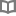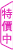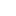大學生一定要做的100件事（三版） - 真敬 - BOOKWALKER中文電子書

大學生一定要做的100件事（三版）

真敬

教育學系講師，曾經擔任多家著名企業的人力管理顧問，目前專職於教學工作。作者對於個人能力的提升與完善，有其獨特的見解。本書就是作者以其豐富的教學經驗，用深入淺出的方式，列舉出大學生必須要做的100件事，希望可以幫助學子們在求學的過程中，透過不斷的學習與成長，加強自己的競爭能力。

• 放入購物車
•試閱• 放入購物車
•試閱• 放入購物車
•試閱• 放入購物車
•試閱• 放入購物車
•試閱• 放入購物車
•試閱• 放入購物車
•試閱• 放入購物車
•試閱• 放入購物車
•試閱• 放入購物車
•試閱• 放入購物車
•試閱• 放入購物車
•試閱• 放入購物車
•試閱• 放入購物車
•試閱• 放入購物車
•試閱• 放入購物車
•試閱

• 放入購物車
•試閱• 放入購物車
•試閱• 放入購物車
•試閱• 放入購物車
•試閱• 放入購物車
•試閱• 放入購物車
•無試閱• 放入購物車
•試閱• 放入購物車
•試閱• 放入購物車
•試閱

• 放入購物車
•試閱

• 放入購物車
•試閱

• 放入購物車
•試閱

• 放入購物車
•試閱• 放入購物車
•試閱

• 放入購物車
•試閱

• 放入購物車
•試閱

• 放入購物車
•試閱

• 放入購物車
•試閱

• 放入購物車
•試閱

• 放入購物車
•試閱

• 放入購物車
•試閱

• 放入購物車
•試閱

• 放入購物車
•試閱

• 放入購物車
•試閱

• 放入購物車
•試閱

• 放入購物車
•試閱